# Algebra II : Sum and Difference of Cubes

## Example Questions

### Example Question #1 : Sum And Difference Of Cubes

Factor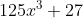.

Possible Answers: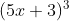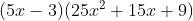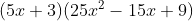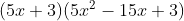Correct answer:Explanation:

The key to solving this problem is noticing that there is something special about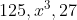; they are all cubes of different values (5, x, 3). Therefore, we can apply the Sum of Cubes formula, which is: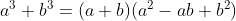In our case, a=5x and b=3. Plugging this in, we get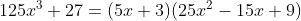Because this is factored, our work here is done! Teachers often encourage students to use the acronym "SOAP" to help them solve Sum and Difference of Cubes problems. The acronym SOAP refers to the signs in the expanded/factored form. The first one is always the Same as in the original equation, the second one is always Opposite the original sign, and the final one is Always Positive no matter. Same, Opposite, Always Positive = SOAP.

### Example Question #1 : Sum And Difference Of Cubes

Factor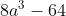Possible Answers: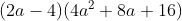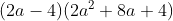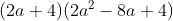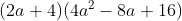Correct answer:Explanation:

The key to solving this problem is noticing that there is something special about 8,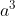, and 64; they are all cubes of different values (2, a, and 4). Therefore, we can apply the Difference of Cubes formula, which is: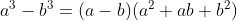In our case, a=2a and b=4. Plugging this in, we get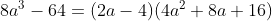Because this is factored, our work here is done! Teachers often encourage students to use the acronym "SOAP" to help them solve Sum and Difference of Cubes problems. The acronym SOAP refers to the signs in the expanded/factored form. The first one is always the Same as in the original equation, the second one is always Opposite the original sign, and the final one is Always Positive no matter. Same, Opposite, Always Positive = SOAP.

### Example Question #2 : Sum And Difference Of Cubes

Factor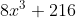.

Possible Answers: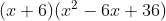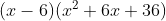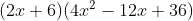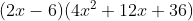Correct answer:Explanation:

The key to solving this problem is noticing that there is something special about 8,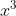, and 216; they are all cubes of different values (2, x, and 6). Therefore, we can apply the Sum of Cubes formula, which is:In our case, a=2x and b=6. Plugging this in, we get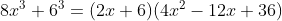Because this is factored, our work here is done! Teachers often encourage students to use the acronym "SOAP" to help them solve Sum and Difference of Cubes problems. The acronym SOAP refers to the signs in the expanded/factored form. The first one is always the Same as in the original equation, the second one is always Opposite the original sign, and the final one is Always Positive no matter. Same, Opposite, Always Positive = SOAP.

### Example Question #3 : Sum And Difference Of Cubes

Which of the following is equivalent to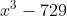?

Possible Answers: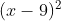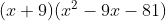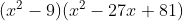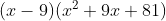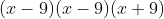Correct answer:Explanation:

Whenever you see the difference of two cubes - as you do here withand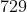, which is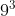, you can apply the formula for Difference of Cubes to factor that term:Here that means you can follow the template with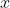and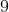to get:### Example Question #5 : Sum And Difference Of Cubes

Which of the following is equivalent to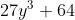?

Possible Answers: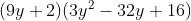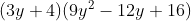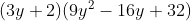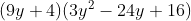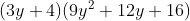Correct answer:Explanation:

Whenever you see the sum of two cubes, as you do here since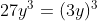and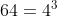, you can factor that sum using the formula: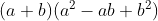Here that allows you to replace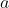with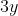and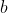with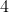to get:### Example Question #6 : Sum And Difference Of Cubes

Which of the following is equivalent to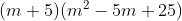?

Possible Answers: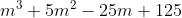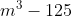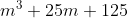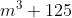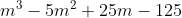Correct answer:Explanation:

This problem has you go backward from a notable rule, the Sum of Cubes rule. You should recognize the Sum of Cubes as.  Here you should see the familiar symptoms of that rule: in the rightmost set of parentheses, you have two squared terms,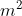and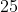, and you have their square roots (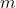and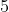) in the leftmost set. Once you've determined that you're dealing with the Sum of Cubes structure, the conversion should be relatively quick without having to distribute the multiplication manually.

### Example Question #4 : Sum And Difference Of Cubes

Which of the following is equivalent to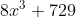?

Possible Answers: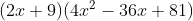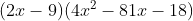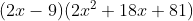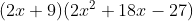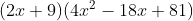Correct answer:Explanation:

This problem employs the Sum of Cubes rule, which states that:Here you should see that the given expression,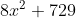, can be written as a sum of two cubes: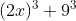. This allows you to invoke the formula, resulting in:### Example Question #4 : Sum And Difference Of Cubes

The expression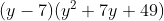is equivalent to:

Possible Answers: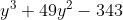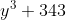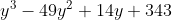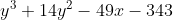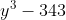Correct answer:Explanation:

This problem calls on your knowledge of the Difference of Cubes rule, which states that:Here you're given two parentheticals in this exact form, allowing you to invoke the rule to solve without manually expanding the parentheses through multiplication. Yourandterms are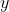and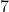, so you can simply express the answer as.

### All Algebra II Resources